# Indexes and arrays: An introduction

Analytica's Intelligent Arrays are central to its power and convenience. They make it much easier and more flexible than spreadsheets and progamming languages to use and build models with multiple dimensions -- such as Time, geographic regioins, products, or scenarios. Even the Run index over random samples is just another dimension. You will find most aspects of Intelligent Arrays natural and intuitive. But you may find some aspects get a little getting used to, especially if you are a spreadsheet expert, or familiar with computer languages, like Visual Basic, R, and Python.

So, even if you usually ignore manuals, we encourage you to you look through this page. It introduces the key concepts of indexes and arrays with a simple example.

### Create an index

An Index identifies a set of options, often used as a dimension of an Array. Suppose you want to compare the fuel cost of three different vehicles, each with different fuel efficiency. We start by defining an index Car_type, which lists three different types of cars as text values. You create a new index, like any new variable, by dragging an index node from the node menu. Once you have created the new node, type in a title Car type.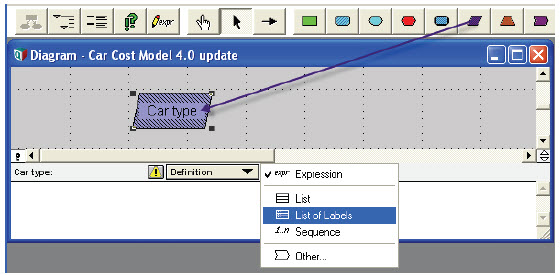Show its definition attribute in the Attribute pane below the diagram, and select List of Labels from the expr menu. Then type the car type the first care type as Standard. Press Enter to add the next cell. Type in Hybrid, and SUV (Sports Utility Vehicle) as two more cells. Now the Car_type is ready to use as an Index for tables, including Edit tables containing input values, and results wiht a value for each each Car type.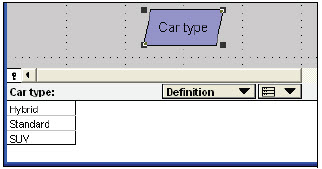### Create an edit table

Create a new variable by dragging it from the node menu, and typing a title Miles per gallon. Draw an arrow from the index Car type to the new node so that you can create a table with numbers for each Car type.

Tip
By default, diagrams don't show arrows to or from index nodes (after you have drawn them.) That's because each index is often used by many other variables. So showing all the arrows tends to clutter up the diagram. But, you can see them if you want. Open the Diagram Style dialog from the Diagram menu, and check Show arrows to/from Indexes .

In the attribute panel above, we show the definition of Miles_per_gallon, and select Table from the expr menu. This opens the Indexes dialog to let you choose which index(es) to use for the table dimensions.It starts with Car_type as the selected index because you drew the arrow from it (see Indexes dialog). Click OK to accept. An edit table appears, indexed by Car_type, with cells initialized to 0.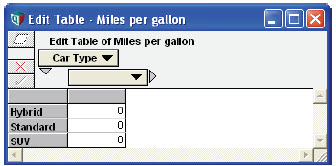You can now edit the cells of the table. Type in a number for each Car_type.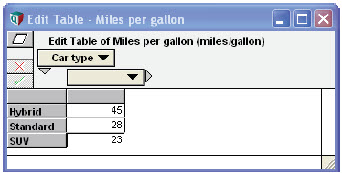This completes the edit table for Miles per gallon.

### Combine a scalar and 1D array

Now let’s calculate the annual fuel cost for each car type. We create three new variables, Miles_per_year, Fuel_price, and Fuel_cost and draw the arrows.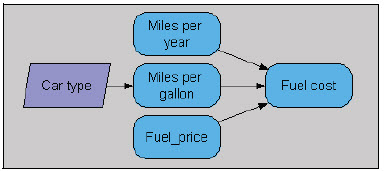Type these definitions for the new variables:

Miles_per_year := 10K
Fuel_price := 3.00
Fuel_cost := Fuel_price * Miles_per_year / Miles_per_gallon

Select Fuel_cost and click the Result button to show this result table.### Array abstraction with operators +, -, *, /

This result for Fuel_cost was computed using Miles_per_gallon for each Car_type, and the single a number, 3.00 for Fuel_price and 10K for Miles_per_year. The arithmetic operators * and / work when one or both operands are arrays, just as well as when they as when they are single number – also known as an atom or scalar . The same is true for +, -, and ^. This is an example of array abstraction, central to Intelligent Arrays™.

### Define another edit table

Now let’s add in the maintenance costs. We create a new variable Maintenance_cost, defined as an edit table, based on the Car_type index, just as we did for Miles_per_gallon.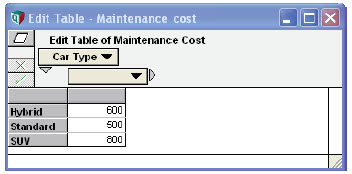We now create Operating_cost as the sum of Fuel_cost and Maintenance_cost. The Diagram looks like this: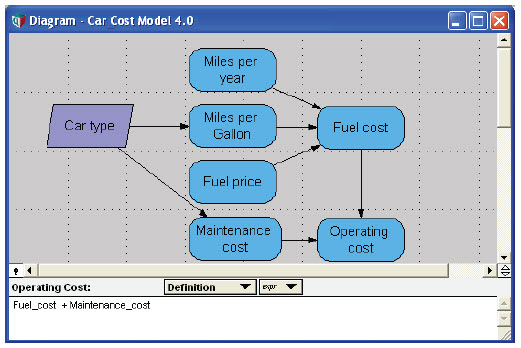Since Fuel_cost and Maintenance_costare both 1D arrays indexed by Car_type, their sum is also indexed by Car_type. Each cell of the result is the simply the sum of the corresponding cells of each input variable: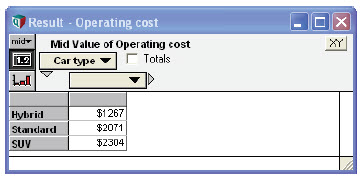### Make an index as a sequence of numbers

Now let’s add another index, Year, so that we can extend the model to compute the costs for multiple years. We create the new index as before. In its definition we enter 2008..2012, to specify the start and end year.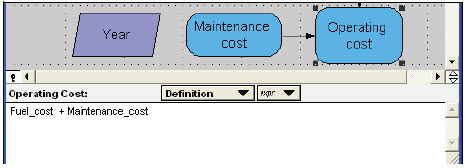The value of Year is now the sequence of years from 2008 to 2012. (See Creating an index and Functions that create indexes for other ways to define indexes.)

Compound annual growth of fuel price by year: What happens if Fuel_price changes over time? Let’s model Fuel_price starting with its current value of 3.00 ($/gallon) multiplied by a compound annual growth rate (CAGR) of 8% per year: Fuel_price_cagr := 8% Fuel_price := 3.00 * (1 + Fuel_price_cagr)^(Year – 2008) This expression says that Fuel_Price starts at 3.0 in Year 2008 (when the exponent (Year - 2008) is zero. For each subsequent year, we raise (1 + 8%) to the power of the number of years from the start year, 2008— i.e., standard compound growth. Here’s the result.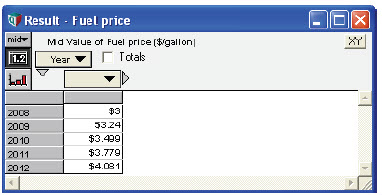Click the graph icon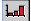to view this as a graph.### Combine two 1D arrays with different indexes Now look at Fuel_cost. Its has three inputs, Miles_per_year, which is still a single number, 10K, Miles_per_gallon, which is indexed by Car_type, and Fuel_price, which is now indexed by Year. The result is a two-dimensional table indexed by both Car_type and Year. It contains every combination of Miles_per_gallon by Car_type and Fuel_price by Year.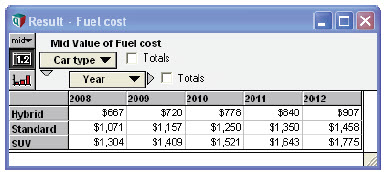### Result has indexes of all operands This illustrates a general rule for Intelligent Arrays: The result of an expression contains the union of the sets of indexes of the elements (operands). Pivot a table, exchanging rows and columns: In the table above, it shows Car_type down the rows and Year across the columns. To pivot the table — i.e., exchange rows and columns — select the other index from the menu defining the columns (or the rows).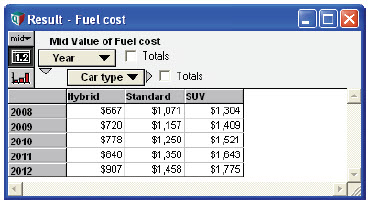(We expanded the window size so that all rows are visible.) ### Rows and columns are just for display of tables Unlike other computer languages, with Analytica, you don’t need to worry about the ordering of the indexes in the table. Rows and columns are simply a question of how you choose to display the table. They are not intrinsic to the internal representation of an array. ### Add a dimension to an edit table Maintenance costs also changes over time, so we need to add Year as dimension. Simply draw an arrow from Year to Maintenance_cost.When it prompts “Do you wish to add Year as a new index of the table in Maintenance_cost?” click Repeat Values. Now open the edit table for Maintenance_cost. It has added Year as a second dimension, copying the number for each Car_type across the years.Notice that it shows the same values for each Year, following the rule that a value is constant over a (previously) unused index. Now you can edit these numbers to reflect how maintenance cost increases over time.### Combine two 2D arrays with the same indexes Let’s look at the value of Operating_cost again.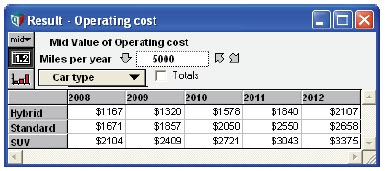Since its inputs, Fuel_cost and Maintenance_cost, are both indexed by Car_type and Year, the result is also indexed by those two indexes. Each cell contains the sum of the corresponding cells from the two input variables. The diagram now looks like this.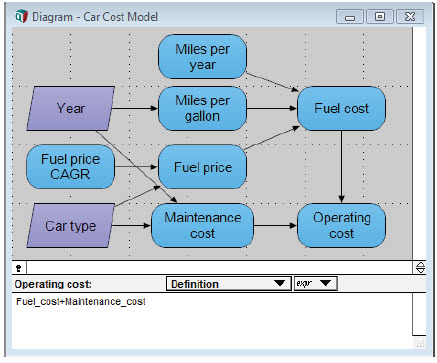### A list of numbers for parametric sensitivity analysis Suppose you’re not sure how many miles you drive per year. You want to examine three scenarios. You include three values in Miles_per_year by specifying a list of numbers enclosed in square brackets: Miles_per_year := [5K, 10K, 15K] Even though Miles_per_year is not defined as an index node, it becomes an implicit index. This is an example of model behavior analysis, described in Parametric analysis. ### Combine three 1D arrays with different indexes Now all three inputs to Fuel_cost are one-dimensional arrays, each with a different index. Its result is a three-dimensional table, computed for each combination of three input variables, so indexed by Miles_per_year, as well as Year and Car_type.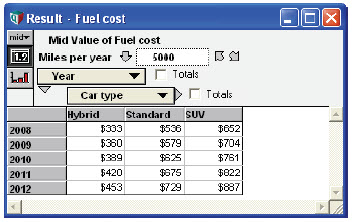The new third index, Miles_per_year, appears as a slicer index, initially showing the slice for 5000 miles/year. You can click the down-arrow for a menu to choose another value, or click the diagonal arrows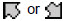to step through the values for miles/year. See Index selection. ### Pivot a 3D table You can also pivot a table to display, for example, the Car_type down the rows and Miles_per_year across the columns, for a selected Year in the slicer.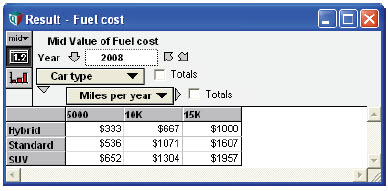### Combine 2D and 3D array with two common indexes When we look at Operating_cost again, it also now has three dimensions. Again the result has the union of the indexes of its operands.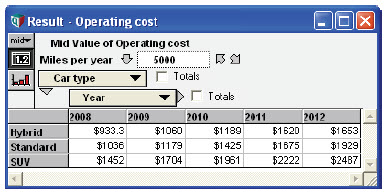It is the sum of fuel cost and maintenance cost, each of which is indexed by Car_type and Year as before, but now Fuel_cost has the third index, Miles_per_year. The result contains all three dimensions. ### Propagate indexes automatically Note how each time we add an index to an input variable, or change a variable, e.g., Miles_per_year, to be a list of values, the new dimensions automatically propagate through the downstream variables. The results have the desired dimensions (the union of the input dimensions) without any need to modify their definitions to mention those indexes explicitly. ### Sum over an index If we want to sum over Year to get the total cost, we define a new variable: Variable Total_operating_cost := Sum(Operating_cost, Year) We mention the index Year, over which we want to calculate the sum. But, we do not need to mention any of the other indexes of the parameter Operating_cost. The built-in function Sum(x, i) is called an array-reducing function, because it reduces its parameter «x» by one dimension, namely «i». There are a variety of other reducing functions, including Max](x, i), Min(x, i), and Product](x, i) (see Array-reducing functions). These functions explicitly specify the index over which they operate. Since they mention it by name, you don’t need to know or worry about any ordering of dimension in the array. ### X[i = v]: subscript The subscript construct lets you extract a slice or subarray from an array, say the values for the Hybrid Car_type: Operating_cost[Car_type = 'Hybrid'] →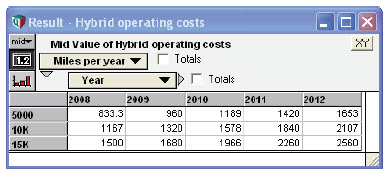You can also select multiple subscripts in one expression: Fuel_cost[year = 2012, Car_type = 'SUV', Miles_per_year = 10K] → 1775 ### Name-based subscripting You can list the indexes in any order since you identify them by name. Again you don’t need to remember which dimension is which. This is called name-based subscripting syntax, in contrast to the more conventional sequence-based subscripting. In addition to absolving you from having to remember the ordering, name-based subscripting generalizes flexibly as you add or remove dimensions of the model. ### Subscripting by an array The value «v» in x[i = v] can itself be an array. For example, if you wanted to get the operating cost only for even years: Operating_cost[Miles_per_year = 10K, Year = [2008, 2010, 2012]]### Purchase price and depreciation To complete the model, let’s add the Purchase_price, an edit table indexed by Car_type (just as we created Miles_per_gallon).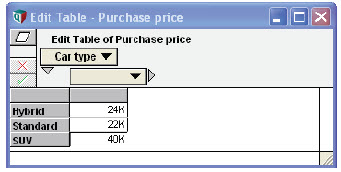To annualize this, we compute the annual depreciation, using a depreciation rate of 18% per year— typical for an automobile: Variable Depreciation_rate := 18% Variable Annual_depreciation := Purchase_price*Depreciation_rate*(1 - Depreciation_rate)^(Year - 2008) It calculates this formula for each Year, raising (1 - Depreciation_rate) to the power of the number of years from 2008.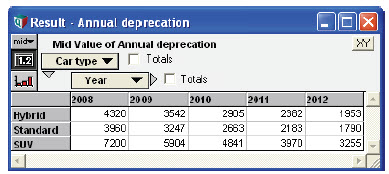### IF THEN ELSE with arrays Suppose that there is a government rebate of$2000 when you purchase a hybrid. You could create an edit table by Car_type and Year with -$2000 for Hybrid in 2008 and $0 in all other cells. (The rebate is negative because we are treating the numbers as costs.) A more elegant method is to define it as a conditional expression based on Year and Car_type:

Variable Hybrid_rebate := IF Year = 2008 AND Car_type = 'Hybrid THEN -2000 ELSE 0

It calculates the expression for each value of the indexes, in this case Year and Car_type, with this result.The subexpression Year = 2008 returns an array indexed by Year containing 1 (true) for 2008 and 0 (false) for the other years. Subexpression Car_type = 'Hybrid'  returns an array indexed by Car_type, containing 1 (true) for 'Hybrid' and 0 (False) for the other Car_type. Therefore, the expression Year = 2008 AND Car_type = 'Hybrid'  returns an array indexed by both Year and Car_type, containing 1 (true) only when both subexpressions are true, that is 1 for Hybrid in 2008 and 0 for the other cells. The entire IF expression therefore returns -2000 for the corresponding top-left cell and 0 for the others.

### Compare a list of variables

To summarize the results, it is useful to compare the four types of cost, Fuel_cost, Maintenance_cost, Purchase_price, and Hybrid_rebate, in one table. Let’s make a variable Cost_summary, and first define it as an empty list, i.e., square brackets with nothing between them yet:

Variable Cost_summary := []

Now draw an arrow from each of the four variables you want to view to Cost_summary, in the sequence you want them to appear. Each time you draw an arrow into a variable defined as a list, it automatically adds that variable into the list. (If the origin variable was already in the list, it removes it again.) Here is the diagram showing the resulting definition for Cost_summary.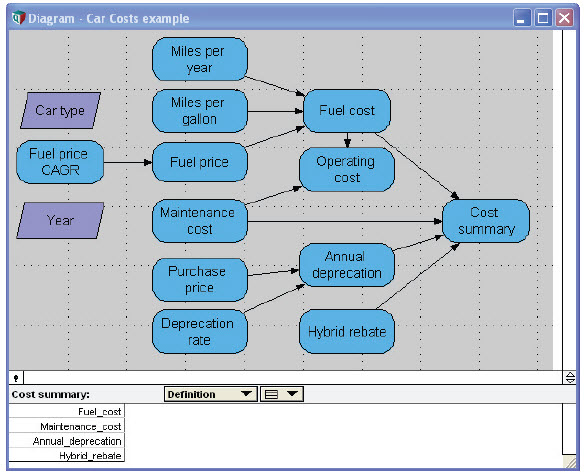Tip
This diagram does not display arrows from index nodes to avoid confusion with crossing arrows. We switched these off by restoring Show arrows to/from Indexes to unchecked (the default) in the Diagram Style dialog from the Diagram menu.

The resulting definition is a list of variables. The result for Cost_summary is four-dimensional, adding a new index, also labeled Cost_summary, showing the variables in the list.### Constant value over an index not in array

Note that only Fuel_cost depends on Miles_per_year. The other three quantities, Maintenance cost, Annual depreciation, and Hybrid rebate, are expanded over that index in the table, using the same number for each value of Miles_per_year. This is an example of a general principle:

• An array that does not contain index «i» as a dimension is treated as though it has the same value over each element of «i» when there is a need to expand it to include «i» as a dimension.

Totals in a table: To see the total over the costs and over the Years, check the two Totals boxes next to the row and column menus.### Self index

The new index containing the titles of the four cost variables in the list is also called Cost_summary. Thus, the identifier Cost_summary serves double-duty as an index for itself. This is known as a self index, and can be accessed using the IndexValue() function.

If we want to compute the sum of the four costs, we can use Sum(x, i) to sum array «x» over index «i». In this case, we sum Cost_summary over its self index, also Cost_summary:

Variable Total_cost_by_year := Sum(Cost_summary, Cost_summary)

### Sum(x, i)

We also want to compute the average cost per mile over all the years. First we compute total cost over time, using the Sum() function:

Variable Total_cost := Sum(Cost_summary, Year)

As before, we need to specify the index over which we are summing, Year, but we don’t need to mention any other indexes, such as Car_type and Miles_per_year, which are irrelevant to this summation.

Next we calculate the Total_miles over Year:

Variable Total_miles := Sum(Miles_per_year, Year)Note that Miles_per_year is not indexed by Year. The principle of Constant value over unused indexes implies that Miles_per_year has the same value for each Year. Hence, the result is the miles per year multiplied the number of years, in this case 5.

Finally, we define:

Variable Cost_per_mile := Total_cost/Total_miles

### Add a new item to an index

What if you want to extend this model to include Compact as a fourth Car_type? Open one of the edit tables indexed by Car_type, say Miles_per_gallon. Click the last Car_type, SUV, to select that row (or column), and press Enter or the down-arrow key. It says “Changing the size of this index will affect table definitions of other variables. Change data in tables indexed by Car_Type?” This warns that adding a new Car_type will affect all the edit tables indexed by Car_type. Click OK, and it adds a new bottom row, with the same label SUV as the previous bot- tom row, and with value 0. Double-click the index label in this bottom row, and type the new Car_type, Compact, to replace it. Then enter its value, say 30 (miles/gallon).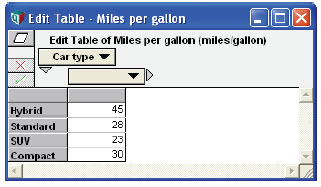### Expanding index for other edit tables

Now open the edit table for Maintenance_cost, and you will see a new row for Compact already added, initialized to 0 in each cell. You just need to enter numbers for Maintenance_cost for the Compact car, as shown here.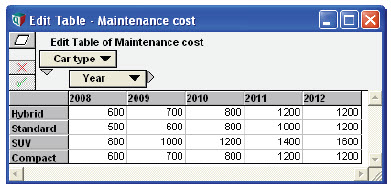Next enter numbers for the Maintenance_cost for the Compact car. Then enter a purchase price for the Compact car.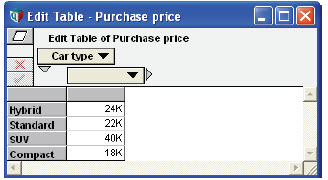### Automatic propagation of changes to index

Now you’ve entered the data for Compact Car_type into the three edit tables, you’re done. All the computed tables automatically inherit the expanded index and do the right thing — without you needing to make any change to their definitions. For example, Cost_summary now looks like this.Finally, let’s compute the net present value cost as the objective, using the reducing function Npv(discount, x, i). We define:

Variable Discount_rate := 12%
Objective NPV_cost := NPV(Discount_rate, Total_cost_by_year, year)

Here is the final diagram, showing NPV_Cost.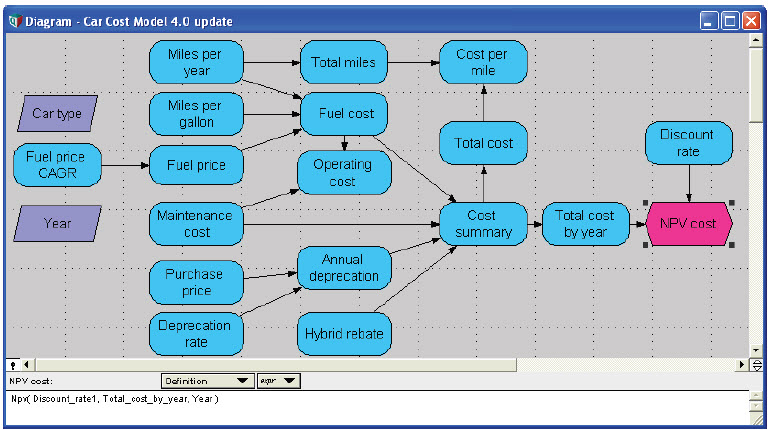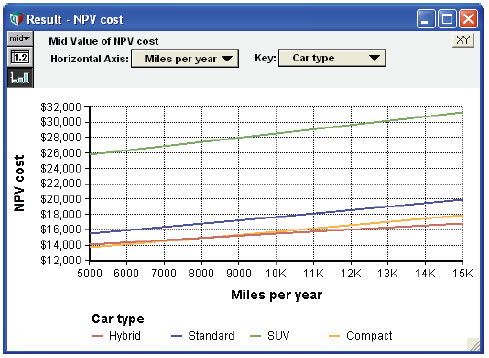### Monte Carlo sampling and Intelligent Arrays

Almost any variable in Analytica can be uncertain — that is, probabilistic. Each probabilistic quantity is represented by a random sample of values, generated using Monte Carlo (or Latin hyper- cube) simulation. Each random sample is an array indexed by a special system variable Run. The value of Run is a sequence of integers from 1 to Sample_size, a system variable specifying the sample size for simulation. For most operations and functions, Run is just another index, and so is handled just like other indexes by the Intelligent Arrays. You can see it when you choose the Sample uncertainty view. In other uncertainty views, such as Mean or CDF, the values displayed are computed from the underlying sample. See Uncertainty views.

### Progressive refinement

As we developed this simple model, we refined it by adding indexes progressively. First, we defined Car_type, then Year, and finally we changed Miles_per_year from a single value to a list of values for parametric analysis. Creating Cost_summary added a fourth index, consisting of the four cost categories. It is often a good idea to build a model like this — starting with a simple version of a model with no or few indexes, and then extending or disaggregating it by adding indexes — and also sometimes removing indexes if they don’t seem important.

This approach to development is sometimes called progressive refinement. By starting simple, you get something working quickly. Then you expand it in steps, adding refinements where they seem to be most useful in improving the representation. A more conventional approach, trying to implement the full detail from the start, risks finding that it’s just too complicated, so it takes a long time to get anything that works. Or, you might find that some of the details are excessive — they just weren’t worth the effort.

Progressive refinement is a much easier in Analytica than in a spreadsheet and most other computer languages — where extending or adding a dimension requires major surgery to the model to add subscripting and loops. With Intelligent Arrays, to extend or add an index, you only need to change edit tables or definitions that actually do something with the new index. The vast majority of formulas generalize appropriately to handle a modified or new dimension without needing any changes.

### Summary of Intelligent Arrays and array abstraction

Intelligent Arrays make it easy to extend and add dimensions (indexes) in Analytica. If you find yourself using a lot of subscripts and explicit iteration with For loops), you might be fighting against Intelligent Arrays rather than letting them do the work for you. Adding unnecessary iteration makes your models harder to write, understand, and maintain. They'll be less robust and will ake much longer to compute than if you let Analytica do its thing. Take the time to understand them, and you should find that you can greatly simplify your model.

In a spreadsheet, extending a dimension means you have (at least) to go through every table that uses that dimension and stretch the cells over the extended rows or columns. It's easy to make mistakes. Adding a dimension is hard work, especially after the first two dimensions. With languages like R or Python, you can easily extend a dimension with new elements, but takes a lot more effort to add a new dimension. Typically, you have to add a loop over the new index and a subscripts to every array variable for every operation. The broadcasting technique in Python libraries can help a bit -- but even there you have to remember the sequencing of indexes, "inner" or "outer", and code carefully to make it all work. In Analytica, each dimension is identified by an Index variable, enabling Analytica to do what you want without you having to even mention the dimensions in most operations, like arithmetic operations, comparisons, and so on.

Almost every operator, construct, and function in Analytica supports array abstraction, automatically generalizing as you add or remove dimensions to their operands or parameters. (See Ensuring Array Abstraction for the few exceptions and how to handle them if you want to make sure that your model fully supports this array abstraction.)

### General principles of Intelligent Arrays™

Omit irrelevant indexes: An expression need not mention any index that it does not explicitly operate over.
A value is constant over unused index: Any function or expression that operates over an Index «i» on a value «x» (atom or array), which does not have «i» as a index, treats «x» as constant over «i» -- i.e. as if it had the same value «x» for each value of «i».
Rows and columns are features of displayed tables, not arrays: You can choose which index to show down the rows or across the columns, or as a slicer dimension. You (almost) never need to think about about the order in which indexes are used in an array.
The indexes of the result of an expression contain the union of the indexes of its component arrays: The result of an operation or expression contains the union of the indexes of any arrays that it uses that is, all indexes from the arrays, without duplicating any index that is in more than one array. There are two unsurprising exceptions:
• When the expression contains an array-reducing function or construct, such as Sum(x, i) or x[i = v], the result will not contain the index «i» over which it is reduced.
• When the expression creates an index, the result will also contain the new index.
To be more precise, we can define the behavior of Intelligent Arrays thus: For any expression or function F(x) that takes a parameter or operand «x» that is an array indexed by «i», for any element «k» of index «i»:
F(x[i = k]) = F(x)[i = k]
In other words, you get the same result if you select element «k» of index «i» of array «x» and then apply F() as if you first apply F() to array «x» and then select element «k» of index «i» of the result. This turns out to be an extremely powerful principle. It underlies Analytica's array abstraction which lets you define expressions on arrays without needing to do explicit iteration over each index as you would need in conventional computer languages.

### Exceptions to array abstraction

The vast majority of operators, constructs, and functions fully support Intelligent Arrays — that is, they generalize appropriately when their operands or parameters are arrays. A few do not, notably the sequence operator (..), Sequence() function, and While loop. When you use these, you need to take special care to ensure that your models perform array abstraction conveniently when you add or modify dimensions. For how, seeEnsuring Array Abstraction.